# Introduction

The parmsurvfit package executes basic parametric survival analysis techniques similar to those in ‘Minitab’. Among these are fitting right-censored data, assessing fit, plotting survival functions, and summary statistics and probabilities.

# Fitting right censored survival data

The fit_data function produces maximum likelihood estimates (MLE) for right censored data based on a specified distribution. Here,

• time: time-to-event variable
• censor: censoring status variable (0 = right-censored; 1 = complete)

Common survival distributions include: Weibull (weibull), log-normal (lnorm), exponential (exp), and logistic (logis).

## Example

library(parmsurvfit)

fit_data(data = firstdrink,
dist = "weibull",
time = "age",
censor = "censor")
#> Fitting of the distribution ' weibull ' on censored data by maximum likelihood
#> Parameters:
#>        estimate
#> shape  2.536106
#> scale 19.684061

# Assessing fit

Assess fit graphically with histograms and overlaid density curves or numerically with the Anderson Darling adjusted test statistic.

## Histograms with density curves

All time to event data are plotted regardless of censoring status.

plot_density(data = firstdrink,
dist = "weibull",
time = "age",
censor = "censor",
by = "gender")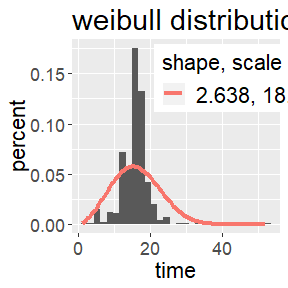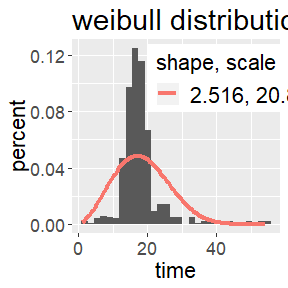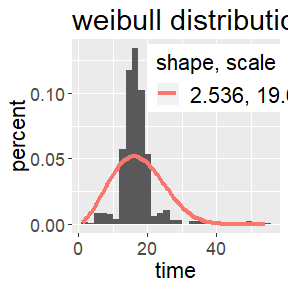## PP-plots

creates a percent-percent plot of right-censored data given that it follows a specified distribution. Points are plotted according to the median rank method to accommodate the right-censored values.

plot_ppsurv(data = firstdrink,
dist = "weibull",
time = "age",
censor = "censor")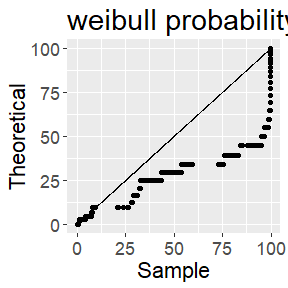## Anderson-Darling test statistic

The Anderson-Darling (AD) test statistic provides a numerical measure of fit such that lower values indicate a better fit. Computation of the test statistic adhered to Minitab’s documentation, utilizing the median rank plotting method.

compute_AD(data = firstdrink,
dist = "weibull",
time = "age",
censor = "censor")
#>  315.5693

# Survival, hazard, and cumulative hazard functions

The survival function $$S(t)$$ estimates the proportion of subjects that survive beyond a specified time $$t$$.

plot_surv(data = firstdrink,
dist = "weibull",
time = "age",
censor = "censor",
by = "gender")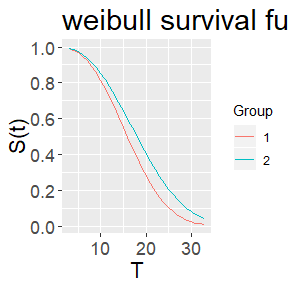The hazard function, denoted $$h(t)$$, estimates the conditional risk that a subject will experience the event of interest in the next instant of time, given that the subject has survived beyond a certain time $$t$$.

plot_haz(data = firstdrink,
dist = "weibull",
time = "age",
censor = "censor",
by = "gender")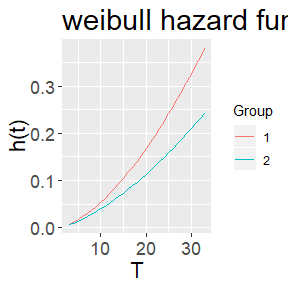The cumulative hazard function, denoted $$H(t)$$, is the total accumulated risk of experiencing an event up to time $$t$$.

plot_cumhaz(data = firstdrink,
dist = "weibull",
time = "age",
censor = "censor",
by = "gender")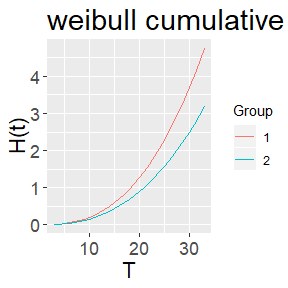# Probabilities and statistics

A survival probability estimates the probability that a subject survives (does not experience the event of interest) beyond a specified time $$t$$.

surv_prob(data = firstdrink,
dist = "weibull",
x = 30,
lower.tail = F,
time = "age",
censor = "censor",
by = "gender")
#>
#> For level = 1
#> P(T > 30) = 0.02488195
#>
#> For level = 2
#> P(T > 30) = 0.08227309
#>
#> For all levels
#> P(T > 30) = 0.05439142

Various summary statistics, including mean, median, standard deviation, and percentiles of survival time. All summary statistics from the class fitdistcens are provided. If the distribution supplied is one of normal, lognormal, exponential, weibull, or logistic then the standard deviation reported is an exact computation from parameter estimates; however, if a user specifies a distribution other than that from this list, then the standard deviation is estimated from 1,000 randomly generated values from the distribution.

surv_summary(data = firstdrink,
dist = "weibull",
time = "age",
censor = "censor",
by = "gender")
#>
#>
#> For level = 1
#> shape        2.637645
#> scale        18.2804
#> Log Liklihood    -1425.271
#> AIC      2854.541
#> BIC      2862.808
#> Mean     16.24398
#> StDev        6.625303
#> First Quantile   11.39844
#> Median       15.90884
#> Third Quantile   20.6903
#>
#> For level = 2
#> shape        2.516025
#> scale        20.85053
#> Log Liklihood    -1730.273
#> AIC      3464.546
#> BIC      3473.126
#> Mean     18.50288
#> StDev        7.872356
#> First Quantile   12.70752
#> Median       18.02407
#> Third Quantile   23.74094